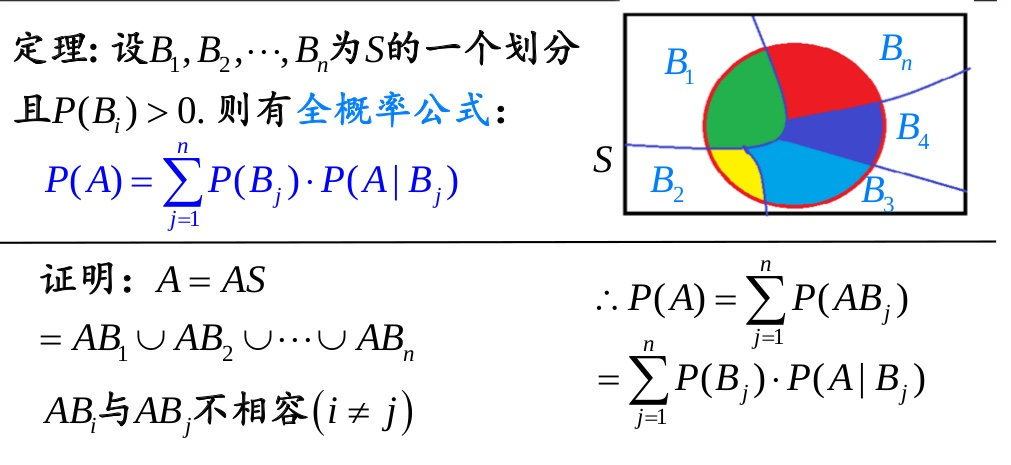## 条件分布

$$P(X=a|Y=b)= \frac{P(X=a,Y=b)}{P(Y=b)}$$

## 独立性

P(X)=P(X|Y)

P(X,Y)=P(X)P(Y)

P(X|Z)=P(X|Y,Z)

P(X,Y|Z)=P(X|Z)P(Y|Z)

## 链式法则

P(X1,X2,…,Xn)=P(X1)P(X2|X1)…P(Xn|X1,X2,…,Xn−1)…………(3)

## 条件概率

#### 条件概率

$$P(A|B)= \frac{P(AB)}{P(B)}$$

P.S. 如果 A，B 是独立事件，则 A 发生的概率与 B 无关，那么 $P(A|B) = P(A)$，并且 $P(AB)=P(A)P(B)$。

#### 联合概率

$P(A\cap B)$ 或者 $P(A,B)$ 或者 $P(A,B)$。

## 全概率公式## 贝叶斯定理

$$P(X|Y)=\frac{P(Y|X)P(X)}{P(Y)}$$